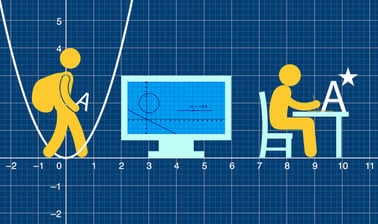## Monday, July 27, 2020

### A-level Mathematics for Year 12 - Course 1: Algebraic Methods, Graphs and Applied Mathematics MethodsThis course by Imperial College London is designed to help you develop the skills you need to succeed in your A-level maths exams.You will investigate key topic areas to gain a deeper understanding of the skills and techniques that you can apply throughout your A-level study. These skills include: Fluency &ndash; selecting and applying correct methods to answer with speed and efficiency Confidence &ndash; critically assessing mathematical methods and investigating ways to apply them Problem solving &ndash; analysing the &lsquo;unfamiliar&rsquo; and identifying which skills and techniques you require to answer questions Constructing mathematical argument &ndash; using mathematical tools such as diagrams, graphs, logical deduction, mathematical symbols, mathematical language, construct mathematical argument and present precisely to others Deep reasoning &ndash; analysing and critiquing mathematical techniques, arguments, formulae and proofs to comprehend how they can be applied Over seven modules, your initial skillset will be extended to give a clear understanding of how background knowledge underpins the A -level course. You&rsquo;ll also be encouraged to consider how what you know fits into the wider mathematical world.